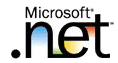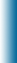Welcome to Mike95.com
 Home Features C++ Library Source Code: COM / ASP Tutorials: Sample Work Internet Based Visual C++ / MFC Java Personal
Algorithms
Euclid's Algorithm
The following algorithm solves a classic elementary problem.
"Reduce a given fraction to its lowest terms", e.g. given 6/9, it returns 2/3.
We sovle this problem by finding the greatest common divisor (gcd) of the numerator and the denominator by using the famous approach called Euclid's algorithm.

First of all, Elucid's algorithm is based completely on the following fact:

If gcd(a,b) is a function which returns the greatest common divisor for both a and b and r is the remainder when a is divided by b, then gcd(a,b)=gcd(b,r).
Having said this, it is now relatively easy to devise a recursive algorithm which follows from this fact.

First we divide the larger number by the smaller number and get the remainder. If the remainder is not 0, then treat the remainder as the "new" small number and the original small number now becomes the "new" large number. Repeate this process with the two new numbers as many times as necessary (usually only a few times) until the remainder becomes 0. Once the remainder is 0, the small number at that point is the lowest common denominator of both original numbers.

 Euclid's Algoritm in C/C++ ```int gcd( int num1, int num2 ) { int remainder = num2 % num1; if ( remainder != 0 ) return gcd( remainder,num1 ); return num1; } ```
Starting this algorithm with gcd(356,96) the following results will occur:
gcd(356,96) = gcd(96,68) = gcd(68,28) = gcd(28,12) = gcd(12,4) = 4
 (c)2022 Mike95.com / Site Disclaimer